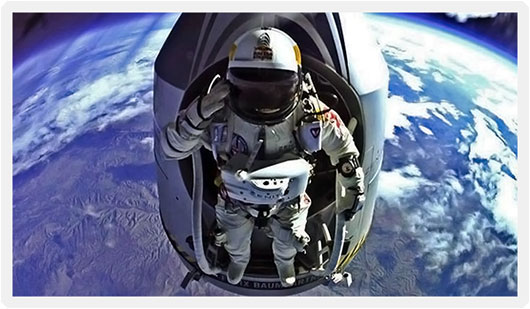# Gravity & Relativity

##It’s All About Predicting Motion

In classical physics, space is a three-dimensional Euclidean space where any position can be described using the three Cartesian coordinates

x, y and z.

The 16th-17th centuries witness Galileo demonstrating that gravitation accelerates all objects at the same rate and Newton hypothesizing the inverse-square law of universal gravitation in 1687.

## Newton’s Laws of Motion and Gravity

Under the influence of the Earth’s mass, any object in freefall experiences a linear downward acceleration due to gravity that is about$g = 9.81 m s^{-2}$

Dropping like a boulder from the tower of Pisa…  The precise magnitude of this acceleration varies according to location, altitude, the effect of Earth’s rotation and variations in its internal composition.

Newton’s Law of Universal Gravitation is$\boldsymbol{F_{grav}}=\frac {-Gm_{1}m_{2}}{r^{2}}$

Measuring the motion of objects relative to one another requires an observer to choose a well-established frame of reference, hence the need for relativity.  Special Relativity use the concept of ‘spacetime’ rather than space.

Spacetime is modelled as a four-dimensional space (with the time axis being imaginary).  Einstein goes further to revolutionize the field with his theory of General Relativity in 1915.  And soon the famous equation makes it into popular culture:$E = mc^2$

The 21st century sees daredevils taking a wild ride on gravity

And the Earth is in constant danger from other projectiles.

A whole new range of exploratory techniques is being developed…

## Little 'Bytes' about Natural Phenomena, Theoretical Physics and the Latest Worldwide Scientific Findings. Edited from Glasgow, Scotland.

NaturPhilosophie, Glasgow, Scotland. Copyright © 2013 - 2019 NaturPhilosophie.co.uk All Rights Reserved. RSS

Privacy Policy